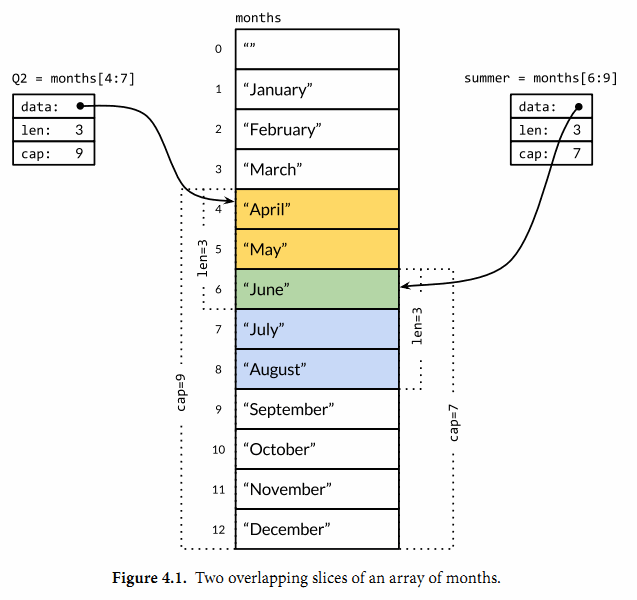## 4.2. Slice

Slice（切片）代表變長的序列，序列中每個元素都有相同的類型。一個slice類型一般寫作[]T，其中T代表slice中元素的類型；slice的語法和數組很像，隻是沒有固定長度而已。

months := [...]string{1: "January", /* ... */, 12: "December"}


slice的切片操作s[i:j]，其中0 ≤ i≤ j≤ cap(s)，用於創建一個新的slice，引用s的從第i個元素開始到第j-1個元素的子序列。新的slice將隻有j-i個元素。如果i位置的索引被省略的話將使用0代替，如果j位置的索引被省略的話將使用len(s)代替。因此，months[1:13]切片操作將引用全部有效的月份，和months[1:]操作等價；months[:]切片操作則是引用整個數組。讓我們分别定義表示第二季度和北方夏天月份的slice，它們有重疊部分：Q2 := months[4:7]
summer := months[6:9]
fmt.Println(Q2)     // ["April" "May" "June"]
fmt.Println(summer) // ["June" "July" "August"]


for _, s := range summer {
for _, q := range Q2 {
if s == q {
fmt.Printf("%s appears in both\n", s)
}
}
}


fmt.Println(summer[:20]) // panic: out of range

endlessSummer := summer[:5] // extend a slice (within capacity)
fmt.Println(endlessSummer)  // "[June July August September October]"


gopl.io/ch4/rev

// reverse reverses a slice of ints in place.
func reverse(s []int) {
for i, j := 0, len(s)-1; i < j; i, j = i+1, j-1 {
s[i], s[j] = s[j], s[i]
}
}


a := [...]int{0, 1, 2, 3, 4, 5}
reverse(a[:])
fmt.Println(a) // "[5 4 3 2 1 0]"


s := []int{0, 1, 2, 3, 4, 5}
// Rotate s left by two positions.
reverse(s[:2])
reverse(s[2:])
reverse(s)
fmt.Println(s) // "[2 3 4 5 0 1]"


func equal(x, y []string) bool {
if len(x) != len(y) {
return false
}
for i := range x {
if x[i] != y[i] {
return false
}
}
return true
}


slice唯一合法的比較操作是和nil比較，例如：

if summer == nil { /* ... */ }


var s []int    // len(s) == 0, s == nil
s = nil        // len(s) == 0, s == nil
s = []int(nil) // len(s) == 0, s == nil
s = []int{}    // len(s) == 0, s != nil


make([]T, len)
make([]T, len, cap) // same as make([]T, cap)[:len]


### 4.2.1. append函數

var runes []rune
for _, r := range "Hello, 世界" {
runes = append(runes, r)
}
fmt.Printf("%q\n", runes) // "['H' 'e' 'l' 'l' 'o' ',' ' ' '世' '界']"


append函數對於理解slice底層是如何工作的非常重要，所以讓我們仔細査看究竟是發生了什麽。下面是第一個版本的appendInt函數，專門用於處理[]int類型的slice：

gopl.io/ch4/append

func appendInt(x []int, y int) []int {
var z []int
zlen := len(x) + 1
if zlen <= cap(x) {
// There is room to grow.  Extend the slice.
z = x[:zlen]
} else {
// There is insufficient space.  Allocate a new array.
// Grow by doubling, for amortized linear complexity.
zcap := zlen
if zcap < 2*len(x) {
zcap = 2 * len(x)
}
z = make([]int, zlen, zcap)
copy(z, x) // a built-in function; see text
}
z[len(x)] = y
return z
}


func main() {
var x, y []int
for i := 0; i < 10; i++ {
y = appendInt(x, i)
fmt.Printf("%d cap=%d\t%v\n", i, cap(y), y)
x = y
}
}


0  cap=1    
1  cap=2    [0 1]
2  cap=4    [0 1 2]
3  cap=4    [0 1 2 3]
4  cap=8    [0 1 2 3 4]
5  cap=8    [0 1 2 3 4 5]
6  cap=8    [0 1 2 3 4 5 6]
7  cap=8    [0 1 2 3 4 5 6 7]
8  cap=16   [0 1 2 3 4 5 6 7 8]
9  cap=16   [0 1 2 3 4 5 6 7 8 9]runes = append(runes, r)


type IntSlice struct {
ptr      *int
len, cap int
}


var x []int
x = append(x, 1)
x = append(x, 2, 3)
x = append(x, 4, 5, 6)
x = append(x, x...) // append the slice x
fmt.Println(x)      // "[1 2 3 4 5 6 1 2 3 4 5 6]"


func appendInt(x []int, y ...int) []int {
var z []int
zlen := len(x) + len(y)
// ...expand z to at least zlen...
copy(z[len(x):], y)
return z
}


### 4.2.2. Slice內存技巧

gopl.io/ch4/nonempty

// Nonempty is an example of an in-place slice algorithm.
package main

import "fmt"

// nonempty returns a slice holding only the non-empty strings.
// The underlying array is modified during the call.
func nonempty(strings []string) []string {
i := 0
for _, s := range strings {
if s != "" {
strings[i] = s
i++
}
}
return strings[:i]
}


data := []string{"one", "", "three"}
fmt.Printf("%q\n", nonempty(data)) // ["one" "three"]
fmt.Printf("%q\n", data)           // ["one" "three" "three"]


nonempty函數也可以使用append函數實現：

func nonempty2(strings []string) []string {
out := strings[:0] // zero-length slice of original
for _, s := range strings {
if s != "" {
out = append(out, s)
}
}
return out
}


stack = append(stack, v) // push v


stack的頂部位置對應slice的最後一個元素：

top := stack[len(stack)-1] // top of stack


stack = stack[:len(stack)-1] // pop


func remove(slice []int, i int) []int {
copy(slice[i:], slice[i+1:])
return slice[:len(slice)-1]
}

func main() {
s := []int{5, 6, 7, 8, 9}
fmt.Println(remove(s, 2)) // "[5 6 8 9]"
}


func remove(slice []int, i int) []int {
slice[i] = slice[len(slice)-1]
return slice[:len(slice)-1]
}

func main() {
s := []int{5, 6, 7, 8, 9}
fmt.Println(remove(s, 2)) // "[5 6 9 8]
}